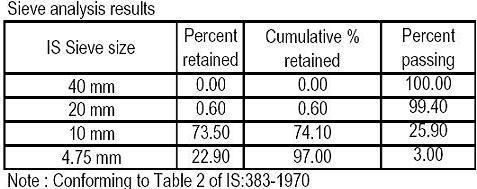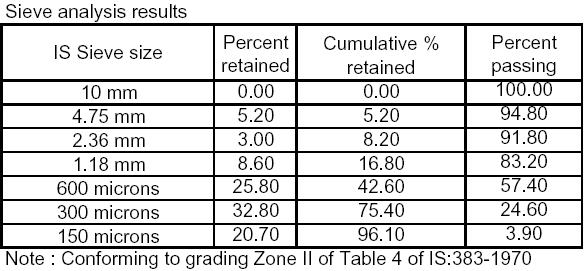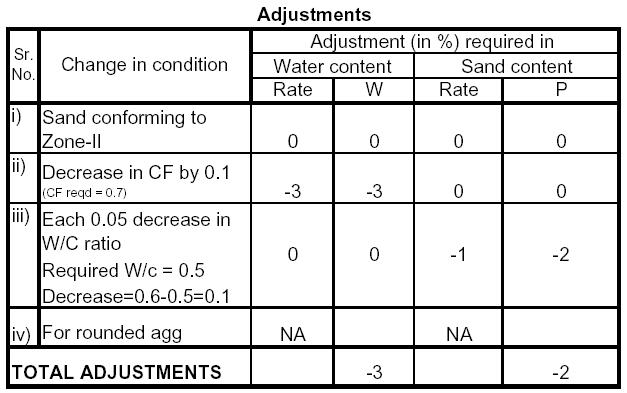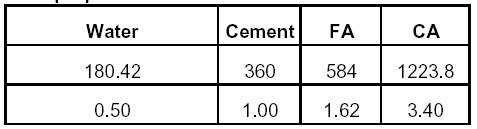Search

# Concrete Mix Design – M 20 Grade Of Concrete

Posted in Mix Design |Email This Post |

1. REQUIREMENTS
a) Specified minimum strength = 20 N/Sq mm

b) Durability requirements
i) Exposure Moderate
ii) Minimum Cement Content = 300 Kgs/cum

c) Cement
(Refer Table No. 5 of IS:456-2000)
i) Make Chetak (Birla)
ii) Type OPC

d) Workability
i) compacting factor = 0.7

e) Degree of quality control Good

2. TEST DATA FOR MATERIALS SUPPLIED
a) CEMENT
i) Specific gravity = 3.05
ii) Avg. comp. strength 7 days = 46.5 more than 33.0 OK
28 days = 55.0 more than 43.0 OK

b) COARSE AGGREGATE
Type Crushed stone aggregate
Specific gravity = 2.68
Water absorption = 1.46
Free (surface) moisture = 0c) FINE AGGREGATE (Coarse sand)
i) Type Natural (Ghaggar)
Specific gravity = 2.6
Water absorption = 0.5
Free (surface) moisture = 1.43. TARGET MEAN STRENGTH (TMS)
a) Statistical constant K = 1.65
b) Standard deviation S = 4.6
Thus, TMS = 27.59 N/Sqmm

4. SELECTION OF W/C RATIO
a) As required for TMS = 0.5
b) As required for ‘Moderate’ Exposure = 0.55
Assume W/c ratio of 0.5

5. DETERMINATION OF WATER & SAND CONTENT
For W/C = 0.6
C.F. = 0.8
Max. Agg. Size of 20 mm
a) Water content = 186 Kg/cum
b) Sand as percentage of total aggregate by absolute volume = 35 %

Thus,
Net water content = 180.42 Kg/cum
Net sand percentage = 33 %6. DETERMINATION OF CEMENT CONTENT
W/c ratio = 0.5
Water content = 180.42 Kg/cum
Thus, Cement content = 360.84 Kg/cum Adequate for moderate exposure Say 360 Kg/cum

7. DETERMINATION OF COARSE AND FINE AGGREGATE CONTENT
Assume entrapped air as 2 %
Thus,
0.98 cum = [180.42+360/3.05 + {1/0.33}*{fa/2.6}]/1000
& 0.98 cum = [180.42+360/3.05 + {1/0.67}*{Ca/2.68}]/1000
Hence,
fa = 584 Kg/cum
Ca = 1223.8 Kg/cum

The final mix proportions of M-20 grade of concrete become:-Note: 1 The above recommended mix design must be verified, by actual cube tests.
2 The mix design is based on the quality and grading of the materials actually supplied, by the client.
Any variation in quality and gradation will result in changes in the mix design.

This mix design was submitted by a regular contributor to this site. We are thankful to him for his excellent service.

More Entries :
• Monali March 27, 2018 at 7:01 pm

What can be the strength of M20 ppc at 28 days?

• ravindra July 1, 2018 at 2:11 am

why is diff bet qty of cement bet pwd and therotical…ex- pwd -m-20-6.9 bag/cu.m…therotical-7.8 bag/cu.m

• Shamshad husain November 13, 2019 at 8:33 am

Sir mix design kyo karte h or kese karte uska slump kese major kare